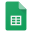# CircuitsReference - Forumla Sheet

## Content Overview

Activities

Ohm on the Range Lab - Search for a relationship between voltage, current, and resistance

An Open & Short Case Lab - Predict which bulbs lose power when you wire them totally wrong

Batteries & Bulbs Lab - Investigate how bulbs work and how well they light up in different configurations

Bread Boards & Resistors Lab - An increasingly challenging set of resistor configurations. Can you solve the puzzles?

Be the Battery Lab - Power different bulbs in series and parallel to feel the difference by hand-cranking a generator

Penguin Power Slider Lab - Put to test all your prior knowledge by finding our how much electrical energy is wasted on a device that sends toys down a slide

Setting (Electrical) Fire to Steel Demo- Fine steel wool & a 9-volt battery demonstrate how a long, thin wire can has high resistance and devastating consequences

Setting (Electrical) Fire to Carbon Demo - A higher voltage and current across pencil "lead" to simulate a light bulb filament and demonstrate high resistance

Bi-directional LED Demo - Direct current in one direction gives a green light, the other red. Is it possible to get both to light up? Yes and no! Introduction to types of currents

Generator & Motor - Crank the generator, spin the motor. Spin the motor, and what happens next? And what happens if we connect a capacitor to it all?

Topics

Voltage

Current

Resistance

Circuit Components when Applicable- switch, resistor, emf, capacitor, diode, etc.

Ohm's Law

Joule's Law

## Students are Expected to Understand

How to wire a circuit in series and in parallel

Techniques involved in measuring voltage and current along a resistor in a circuit

Plan, create, and interpret circuit diagrams

Predict and calculate voltage, current, resistance, and power in a circuit

### Some Notes on Circuits

• Batteries can come in many shapes and sizes, but those qualities don't necessarily affect the voltage of a household battery as voltage is primarily affected by internal chemistry
• Voltage is the energy of the charges in the circuit
• Current is the flow rate or the number of charges moving through in the circuit
• Current for resistors in series is constant
• Resistors in series split up the available voltage among the resistors with the biggest resistor getting the most voltage
• Resistors soak up energy in the circuit and can be used to change current, voltage, and power, and are oftentimes used to transform energy in a circuit into different things like heat, light, sound, or motion
• Resistors can be thought of as straws and current as air passing through that straw. The longer the straw and the thinner the straw the harder it is to push air through. A thin, long wire will also have a lot of resistance. Sometimes, there's so much resistance that the wire gets really hot and catches things near it on fire!
• A short circuit can bypass resistors and may case problems in a circuit, like overloading and damaging sensitive parts with too much current or overheating it and catching fire
• Voltage for resistors in parallel is constant
• Resistors in parallel split up the available current among the resistors with a higher current passing through the smallest resistor
• Joule's Law (P = IV )and Ohm's Law (V = IR) can be substituted into each other to solve for any two unknown variables given the other two variables

Ever since we've discovered that we can put moving charges to work, we've been using electricity and circuits in an increasing number of applications. This unit will cover how electricity behaves, manipulation of circuits, and determining circuit outcomes.

*These videos are to be used as a supplement and are not a replacement for in-class experiences

# Circuit Diagram Symbol Guide# Series Circuit Example

See if you can find the following:

• Total resistance in series, Rseries Rseries = R1 + R2 + R3; 50 Ω = 10 Ω + 15 Ω + 25 Ω
• The current in the circuit, I V = IR; I = V/R; 0.1 A = 4 V / 14 Ω
• Voltage V1 along R1 V1 = IR1; 0.1 V1 = 0.1 A x 10 Ω
• Voltage V2 along R2 V2 = IR2; 0.15 V2 = 0.1 A x 15 Ω
• Voltage V3 along R3 V3 = IR3; 0.25 V3 = 0.1 A x 25 Ω# Parallel Circuit Example

See if you can find the following knowing that Vbattery = 6 V, R1 = 12 Ω, R2 = 24 Ω

• Total resistance in parallel, 1/Rparallel 1/Rparallel = 1/R1 + 1/R2

1/R = 1/12 Ω + 1/24 Ω = 3/24 Ω = 24 Ω/3 = 8 Ω

• The current in the circuit V = IR; I = V/R 1.5 A = 6 V / 8 Ω
• Current I1 along R1 I1 = V1/R1 0.5 A = 6 V / 12 Ω
• Current I2 along R2 I2 = V2/R2 6 V / 24 Ω